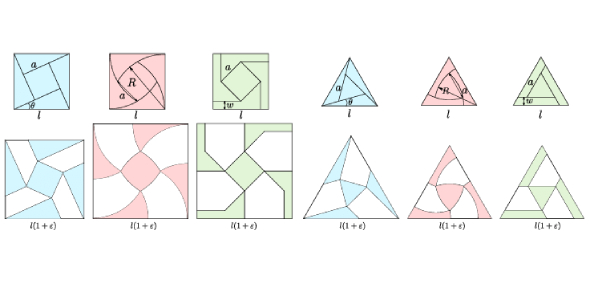# Building Blocks Of Geometry Quiz! Test

Approved & Edited by ProProfs Editorial Team
At ProProfs Quizzes, our dedicated in-house team of experts takes pride in their work. With a sharp eye for detail, they meticulously review each quiz. This ensures that every quiz, taken by over 100 million users, meets our standards of accuracy, clarity, and engagement.
| Written by Dfitzpatrick
D
Dfitzpatrick
Community Contributor
Quizzes Created: 10 | Total Attempts: 2,612
Questions: 10 | Attempts: 175SettingsWhat can be said about the building blocks of geometry? Geometry is one of the most renowned forms of mathematics. It is involved with space-related properties to distance, shape, size, and relative position of items. Since its inception, geometry has been divided into many subcategories that rely on specific methods. Geometry has applications in all forms of science. This quiz will help to shape you as a winner.

• 1.

### "The ray through point P from point Q" is written in symbolic for as .

• A.

True

• B.

False

B. False
Explanation
It is written as

Rate this question:

• 2.

### "The length of segment PQ" can be written as PQ.

• A.

True

• B.

False

A. True
Explanation
The statement is true because the length of segment PQ can indeed be represented as PQ. In geometry, segments are typically labeled using two points, in this case, P and Q. The length of a segment is denoted by the distance between these two points, which can be represented as PQ.

Rate this question:

• 3.

### The vertex of angle PDQ is point P.

• A.

True

• B.

False

B. False
Explanation
The vertex is point D.

Rate this question:

• 4.

### The symbol for perpendicular is .

• A.

True

• B.

False

A. True
Explanation
The symbol for perpendicular is a right angle symbol, which looks like a small square placed at the vertex of an upside-down capital letter "T". This symbol is commonly used in geometry to represent two lines or line segments that intersect at a 90-degree angle. Therefore, the given statement is true.

Rate this question:

• 5.

### A scalene triangle is a triangle with no two sides the same length.

• A.

True

• B.

False

A. True
Explanation
A scalene triangle is a type of triangle where all three sides have different lengths. This means that no two sides of the triangle are the same length. Therefore, the statement "A scalene triangle is a triangle with no two sides the same length" is true.

Rate this question:

• 6.

### An acute angle is an angle whose measure is more than .

• A.

True

• B.

False

B. False
Explanation
Acute angles measure less than 90 degrees.

Rate this question:

• 7.

### If  intersects  at point P, then  and  are a pair of vertical angles.

• A.

True

• B.

False

B. False
Explanation
Vertical angles are formed when two lines intersect. In this case, the question does not provide any information about lines intersecting. Therefore, we cannot determine if the angles are vertical or not. Hence, the correct answer is False.

Rate this question:

• 8.

### A diagonal is a line segment in a polygon connecting any two nonconsecutive vertices.

• A.

True

• B.

False

A. True
Explanation
A diagonal is a line segment that connects two nonconsecutive vertices in a polygon. This means that it does not connect two adjacent vertices. Therefore, the statement that a diagonal is a line segment in a polygon connecting any two nonconsecutive vertices is true.

Rate this question:

• 9.

### If the sum of the measures of two angles is , then the two angles are complementary.

• A.

True

• B.

FalseBack to top